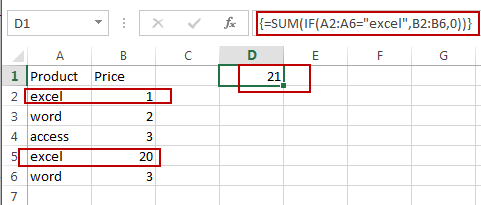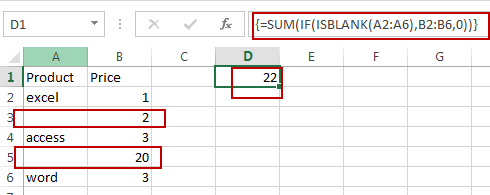# How to SUM Cells If the Adjacent Cell Match One Criteria in Excel

This post will guide you how to sum a given range of cells that if the adjacent cell meet one criteria in Excel. How do I sum All Cells if its adjacent cell is blank or equals to zero or contain a specific text string value with a formula in Excel.

## SUM Cells If the Adjacent Cell Match One Criteria

Assuming that you have a list of data in range A1:B5, in which contain sales data.  You want to sum all sales values where the adjacent cell equal to product “excel”. How to do it. You can use an Excel Array formula based on the SUM function and the IF function to achieve the result. Like this:

`=SUM(IF(A2:A6="excel",B2:B6,0))`

You need to type this formula into a blank cell and press Ctrl + Alt + Enter keys on your keyboard to change the normal formula as array formula.Note: the A2:A6 range is the data range which contain the criteria that need to match. And the B2:B6 is a range which have sales values that you want to sum based on the matched criteria.

If you want to sum all values if the adjacent cell is a blank cell in your data, you can use another array formula based on the SUM function, the IF function and the ISBLANK function to achieve the result. Like this:

`=SUM(IF(ISBLANK(A2:A6),B2:B6,0))`

Type this formula into a blank cell and press Ctrl + Alt + Enter keys on your keyboard.

### Related Functions

• Excel SUM function
The Excel SUM function will adds all numbers in a range of cells and returns the sum of these values. You can add individual values, cell references or ranges in excel.The syntax of the SUM function is as below:= SUM(number1,[number2],…)…
• Excel IF function
The Excel IF function perform a logical test to return one value if the condition is TRUE and return another value if the condition is FALSE. The IF function is a build-in function in Microsoft Excel and it is categorized as a Logical Function.The syntax of the IF function is as below:= IF (condition, [true_value], [false_value])….
• Excel ISBLANK function
The Excel ISBLANK function returns TRUE if the value is blank or null.The syntax of the ISBLANK function is as below:= ISBLANK (value)…